# 87 Arithmetic Sequences

### Learning Objectives

In this section, you will:

• Find the common difference for an arithmetic sequence.
• Write terms of an arithmetic sequence.
• Use a recursive formula for an arithmetic sequence.
• Use an explicit formula for an arithmetic sequence.

Companies often make large purchases, such as computers and vehicles, for business use. The book-value of these supplies decreases each year for tax purposes. This decrease in value is called depreciation. One method of calculating depreciation is straight-line depreciation, in which the value of the asset decreases by the same amount each year.

### Try It

A woman decides to go for a 10-minute run every day this week and plans to increase the time of her daily run by 4 minutes each week. Write a formula for the time of her run after n weeks. How long will her daily run be 8 weeks from today?

The formula is ${T}_{n}=10+4n,\,$and it will take her 42 minutes.

Access this online resource for additional instruction and practice with arithmetic sequences.

### Key Equations

 recursive formula for nth term of an arithmetic sequence ${a}_{n}={a}_{n-1}+d,n\ge 2$ explicit formula for nth term of an arithmetic sequence $\begin{array}{l}{a}_{n}={a}_{1}+d\left(n-1\right)\end{array}$

### Key Concepts

• An arithmetic sequence is a sequence where the difference between any two consecutive terms is a constant.
• The constant between two consecutive terms is called the common difference.
• The common difference is the number added to any one term of an arithmetic sequence that generates the subsequent term. See (Figure).
• The terms of an arithmetic sequence can be found by beginning with the initial term and adding the common difference repeatedly. See (Figure) and (Figure).
• A recursive formula for an arithmetic sequence with common difference $d$ is given by ${a}_{n}={a}_{n-1}+d,n\ge 2.$ See (Figure).
• As with any recursive formula, the initial term of the sequence must be given.
• An explicit formula for an arithmetic sequence with common difference $d$ is given by ${a}_{n}={a}_{1}+d\left(n-1\right).$ See (Figure).
• An explicit formula can be used to find the number of terms in a sequence. See (Figure).
• In application problems, we sometimes alter the explicit formula slightly to ${a}_{n}={a}_{0}+dn.$ See (Figure).

### Section Exercises

#### Verbal

What is an arithmetic sequence?

A sequence where each successive term of the sequence increases (or decreases) by a constant value.

How is the common difference of an arithmetic sequence found?

How do we determine whether a sequence is arithmetic?

[hidden-answer a=”321648″]We find whether the difference between all consecutive terms is the same. This is the same as saying that the sequence has a common difference.[/hidden-answer]

What are the main differences between using a recursive formula and using an explicit formula to describe an arithmetic sequence?

Describe how linear functions and arithmetic sequences are similar. How are they different?

Both arithmetic sequences and linear functions have a constant rate of change. They are different because their domains are not the same; linear functions are defined for all real numbers, and arithmetic sequences are defined for natural numbers or a subset of the natural numbers.

#### Algebraic

For the following exercises, find the common difference for the arithmetic sequence provided.

$\left\{5,11,17,23,29,...\right\}$

$\left\{0,\frac{1}{2},1,\frac{3}{2},2,...\right\}$

The common difference is $\frac{1}{2}$

For the following exercises, determine whether the sequence is arithmetic. If so find the common difference.

$\left\{11.4,9.3,7.2,5.1,3,...\right\}$

$\left\{4,16,64,256,1024,...\right\}$

The sequence is not arithmetic because $16-4\ne 64-16.$

For the following exercises, write the first five terms of the arithmetic sequence given the first term and common difference.

${a}_{1}=-25$, $d=-9$

${a}_{1}=0$, $d=\frac{2}{3}$

$0,\,\frac{2}{3},\,\frac{4}{3},\,2,\,\frac{8}{3}$

For the following exercises, write the first five terms of the arithmetic series given two terms.

${a}_{1}=17,\,{a}_{7}=-31$

${a}_{13}=-60,\,{a}_{33}=-160$

$0,-5,-10,-15,-20$

For the following exercises, find the specified term for the arithmetic sequence given the first term and common difference.

First term is 3, common difference is 4, find the 5th term.

First term is 4, common difference is 5, find the 4th term.

${a}_{4}=19$

First term is 5, common difference is 6, find the 8th term.

First term is 6, common difference is 7, find the 6th term.

${a}_{6}=41$

First term is 7, common difference is 8, find the 7th term.

For the following exercises, find the first term given two terms from an arithmetic sequence.

Find the first term or ${a}_{1}$ of an arithmetic sequence if ${a}_{6}=12$ and ${a}_{14}=28.$

${a}_{1}=2$

Find the first term or ${a}_{1}$ of an arithmetic sequence if ${a}_{7}=21$ and ${a}_{15}=42.\,$

Find the first term or ${a}_{1}$ of an arithmetic sequence if ${a}_{8}=40$ and ${a}_{23}=115.$

${a}_{1}=5$

Find the first term or ${a}_{1}$ of an arithmetic sequence if ${a}_{9}=54$ and ${a}_{17}=102.$

Find the first term or ${a}_{1}$ of an arithmetic sequence if ${a}_{11}=11$ and ${a}_{21}=16.$

${a}_{1}=6$

For the following exercises, find the specified term given two terms from an arithmetic sequence.

${a}_{1}=33\,$and$\,{a}_{7}=-15.$ Find$\,{a}_{4}.$

${a}_{3}=-17.1\,$and$\,{a}_{10}=-15.7.$ Find${a}_{21}.$

${a}_{21}=-13.5$

For the following exercises, use the recursive formula to write the first five terms of the arithmetic sequence.

${a}_{1}=39;\text{ }{a}_{n}={a}_{n-1}-3$

${a}_{1}=-19;\text{ }{a}_{n}={a}_{n-1}-1.4$

$-19,-20.4,-21.8,-23.2,-24.6$

For the following exercises, write a recursive formula for each arithmetic sequence.

${a}_{n}=\left\{40,60,80,...\right\}$

${a}_{n}=\left\{17,26,35,...\right\}$

$\begin{array}{ll}{a}_{1}=17; {a}_{n}={a}_{n-1}+9\hfill & n\ge 2\hfill \end{array}$

${a}_{n}=\left\{-1,2,5,...\right\}$

${a}_{n}=\left\{12,17,22,...\right\}$

$\begin{array}{ll}{a}_{1}=12; {a}_{n}={a}_{n-1}+5\hfill & n\ge 2\hfill \end{array}$

${a}_{n}=\left\{-15,-7,1,...\right\}$

${a}_{n}=\left\{8.9,10.3,11.7,...\right\}$

$\begin{array}{ll}{a}_{1}=8.9; {a}_{n}={a}_{n-1}+1.4\hfill & n\ge 2\hfill \end{array}$[/hidden-answer]

${a}_{n}=\left\{-0.52,-1.02,-1.52,...\right\}$

${a}_{n}=\left\{\frac{1}{5},\frac{9}{20},\frac{7}{10},...\right\}$

$\begin{array}{ll}{a}_{1}=\frac{1}{5}; {a}_{n}={a}_{n-1}+\frac{1}{4}\hfill & n\ge 2\hfill \end{array}$

${a}_{n}=\left\{-\frac{1}{2},-\frac{5}{4},-2,...\right\}$

${a}_{n}=\left\{\frac{1}{6},-\frac{11}{12},-2,...\right\}$

$\begin{array}{ll}{}_{1}=\frac{1}{6}; {a}_{n}={a}_{n-1}-\frac{13}{12}\hfill & n\ge 2\hfill \end{array}$

For the following exercises, write a recursive formula for the given arithmetic sequence, and then find the specified term.

${a}_{n}=\left\{7\text{, }4\text{, }1\text{, }...\right\};\,$Find the 17th term.

${a}_{n}=\left\{4\text{, }11\text{, }18\text{, }...\right\};\,$Find the 14th term.

${a}_{1}=4;\text{ }{a}_{n}={a}_{n-1}+7;\text{ }{a}_{14}=95$

${a}_{n}=\left\{2\text{, }6\text{, }10\text{, }...\right\};\,$Find the 12th term.

For the following exercises, use the explicit formula to write the first five terms of the arithmetic sequence.

${a}_{n}=24-4n$

[hidden-answer a=”fs-id1165135194498″]First five terms: $20,16,12,8,4.$[/hidden-answer]

${a}_{n}=\frac{1}{2}n-\frac{1}{2}$

For the following exercises, write an explicit formula for each arithmetic sequence.

${a}_{n}=\left\{3,5,7,...\right\}$

${a}_{n}=1+2n$

${a}_{n}=\left\{32,24,16,...\right\}$

${a}_{n}=\left\{-5\text{, }95\text{, }195\text{, }...\right\}$

${a}_{n}=-105+100n$[/hidden-answer]

${a}_{n}=\left\{-17\text{, }-217\text{, }-417\text{,}...\right\}$

${a}_{n}=\left\{1.8\text{, }3.6\text{, }5.4\text{, }...\right\}$

${a}_{n}=1.8n$

${a}_{n}=\left\{-18.1,-16.2,-14.3,...\right\}$

${a}_{n}=\left\{15.8,18.5,21.2,...\right\}$

${a}_{n}=13.1+2.7n$

${a}_{n}=\left\{\frac{1}{3},-\frac{4}{3},-3\text{, }...\right\}$

${a}_{n}=\left\{0,\frac{1}{3},\frac{2}{3},...\right\}$

${a}_{n}=\frac{1}{3}n-\frac{1}{3}$

${a}_{n}=\left\{-5,-\frac{10}{3},-\frac{5}{3},\dots \right\}$

For the following exercises, find the number of terms in the given finite arithmetic sequence.

${a}_{n}=\left\{3\text{,}-4\text{,}-11\text{, }...\text{,}-60\right\}$

There are 10 terms in the sequence.

${a}_{n}=\left\{1.2,1.4,1.6,...,3.8\right\}$

${a}_{n}=\left\{\frac{1}{2},2,\frac{7}{2},...,8\right\}$

There are 6 terms in the sequence.

#### Graphical

For the following exercises, determine whether the graph shown represents an arithmetic sequence.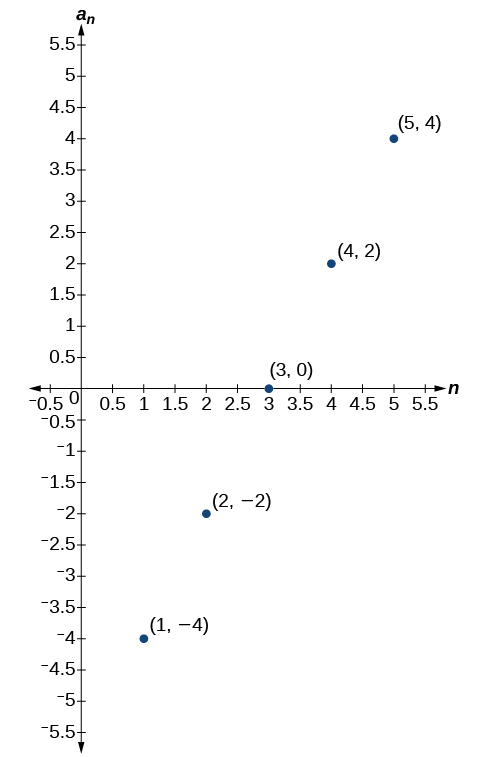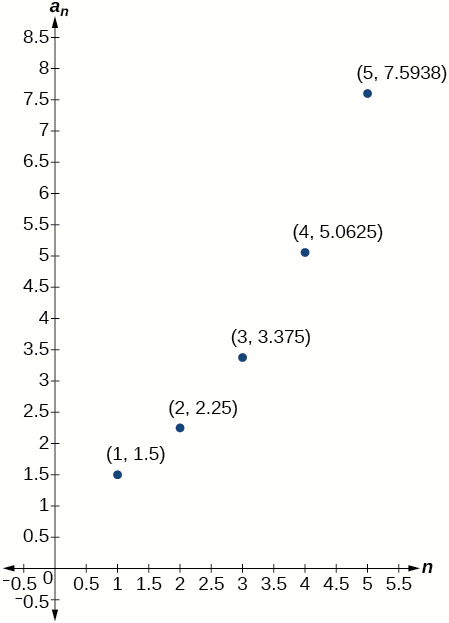The graph does not represent an arithmetic sequence.

For the following exercises, use the information provided to graph the first 5 terms of the arithmetic sequence.

${a}_{1}=0,d=4$

${a}_{1}=9;{a}_{n}={a}_{n-1}-10$

[hidden-answer a=”fs-id1165137532662″]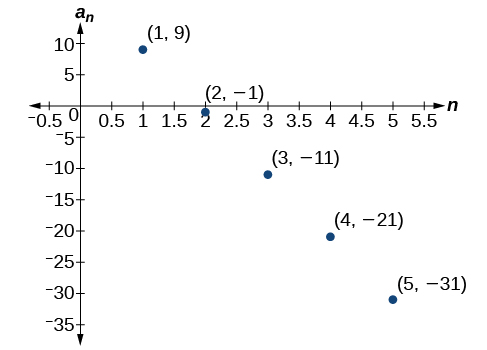[/hidden-answer]

${a}_{n}=-12+5n$

#### Technology

For the following exercises, follow the steps to work with the arithmetic sequence ${a}_{n}=3n-2$ using a graphing calculator:

• Press [MODE]
• Select SEQ in the fourth line
• Select DOT in the fifth line
• Press [ENTER]
• Press [Y=]
• $n\text{Min}\,$is the first counting number for the sequence. Set $\,n\text{Min}=1$
• $u\left(n\right)\,$is the pattern for the sequence. Set $\,u\left(n\right)=3n-2$
• $u(n\text{Min)}\,$is the first number in the sequence. Set $\,u(n\text{Min)}=1$
• Press [2ND] then [WINDOW] to go to TBLSET
• Set$\,\text{TblStart}=1$
• Set$\,\Delta \text{Tbl}=1$
• Set Indpnt: Auto and Depend: Auto
• Press [2ND] then [GRAPH] to go to the TABLE

What are the first seven terms shown in the column with the heading $u\left(n\right)\text{?}$

$1,4,7,10,13,16,19$

Use the scroll-down arrow to scroll to$n=50.$ What value is given for $u\left(n\right)\text{?}$

Press [WINDOW]. Set$\,n\text{Min}=1,n\text{Max}=5,x\text{Min}=0,x\text{Max}=6,y\text{Min}=-1,\,$and$\,y\text{Max}=14.\,$Then press [GRAPH]. Graph the sequence as it appears on the graphing calculator.

[hidden-answer a=”fs-id1165137828039″]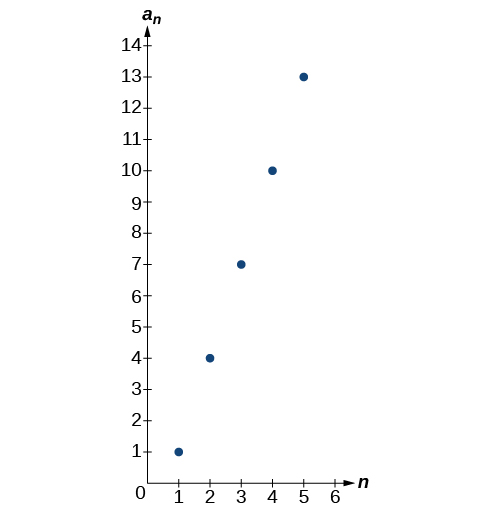[/hidden-answer]

For the following exercises, follow the steps given above to work with the arithmetic sequence ${a}_{n}=\frac{1}{2}n+5$ using a graphing calculator.

What are the first seven terms shown in the column with the heading$\,u\left(n\right)\,$in the TABLE feature?

Graph the sequence as it appears on the graphing calculator. Be sure to adjust the WINDOW settings as needed.

[hidden-answer a=”fs-id1165135307896″]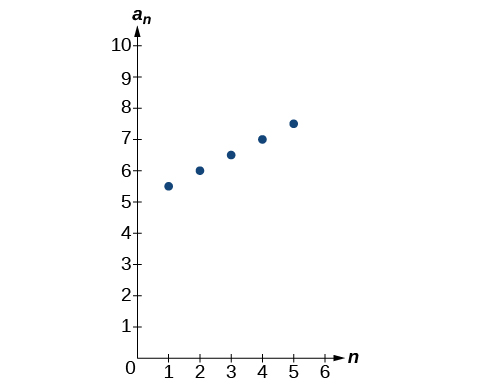[/hidden-answer]

#### Extensions

Give two examples of arithmetic sequences whose 4th terms are $9.$

Give two examples of arithmetic sequences whose 10th terms are $206.$

Answers will vary. Examples: ${a}_{n}=20.6n$and${a}_{n}=2+20.4\mathrm{n.}$

Find the 5th term of the arithmetic sequence $\left\{9b,5b,b,\dots \right\}.$

Find the 11th term of the arithmetic sequence $\left\{3a-2b,a+2b,-a+6b\dots \right\}.$

${a}_{11}=-17a+38b$

At which term does the sequence $\left\{5.4,14.5,23.6,...\right\}$ exceed 151?

At which term does the sequence $\left\{\frac{17}{3},\frac{31}{6},\frac{14}{3},...\right\}$ begin to have negative values?

The sequence begins to have negative values at the 13th term, ${a}_{13}=-\frac{1}{3}$

For which terms does the finite arithmetic sequence $\left\{\frac{5}{2},\frac{19}{8},\frac{9}{4},...,\frac{1}{8}\right\}$ have integer values?

Write an arithmetic sequence using a recursive formula. Show the first 4 terms, and then find the 31st term.

Answers will vary. Check to see that the sequence is arithmetic. Example: Recursive formula: ${a}_{1}=3,{a}_{n}={a}_{n-1}-3.$ First 4 terms: $\begin{array}{ll}3,0,-3,-6\hfill & {a}_{31}=-87\hfill \end{array}$

Write an arithmetic sequence using an explicit formula. Show the first 4 terms, and then find the 28th term.

### Glossary

arithmetic sequence
a sequence in which the difference between any two consecutive terms is a constant
common difference
the difference between any two consecutive terms in an arithmetic sequence